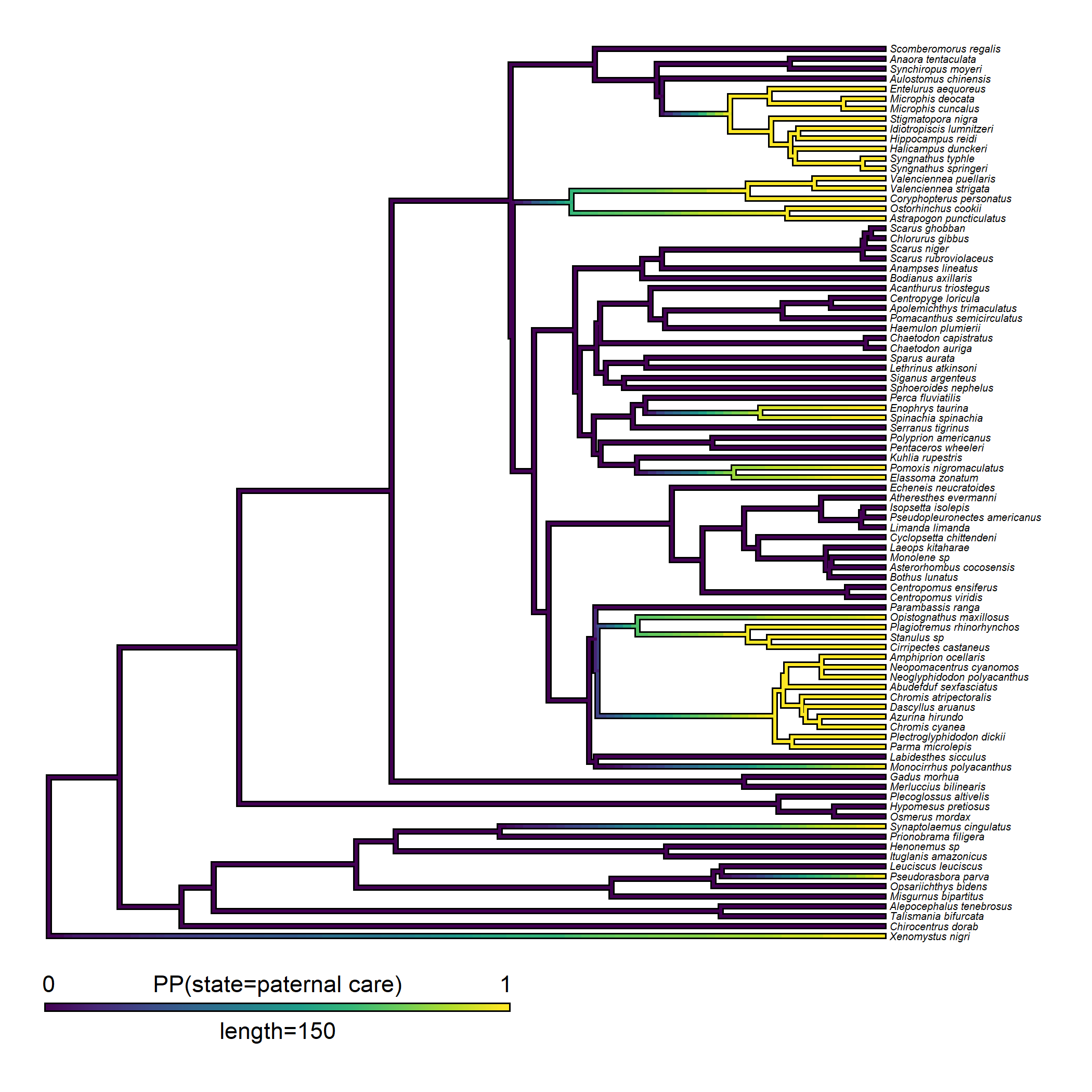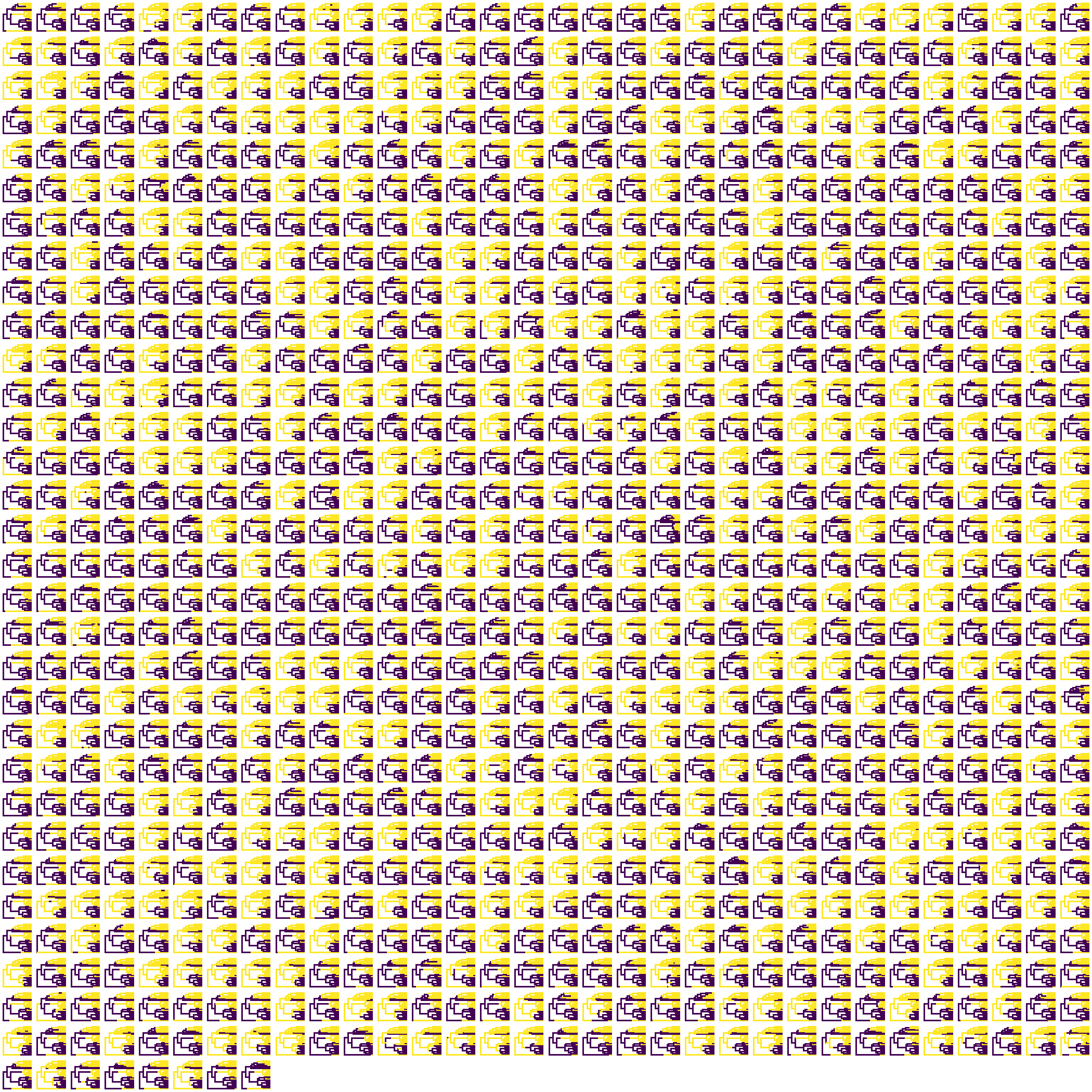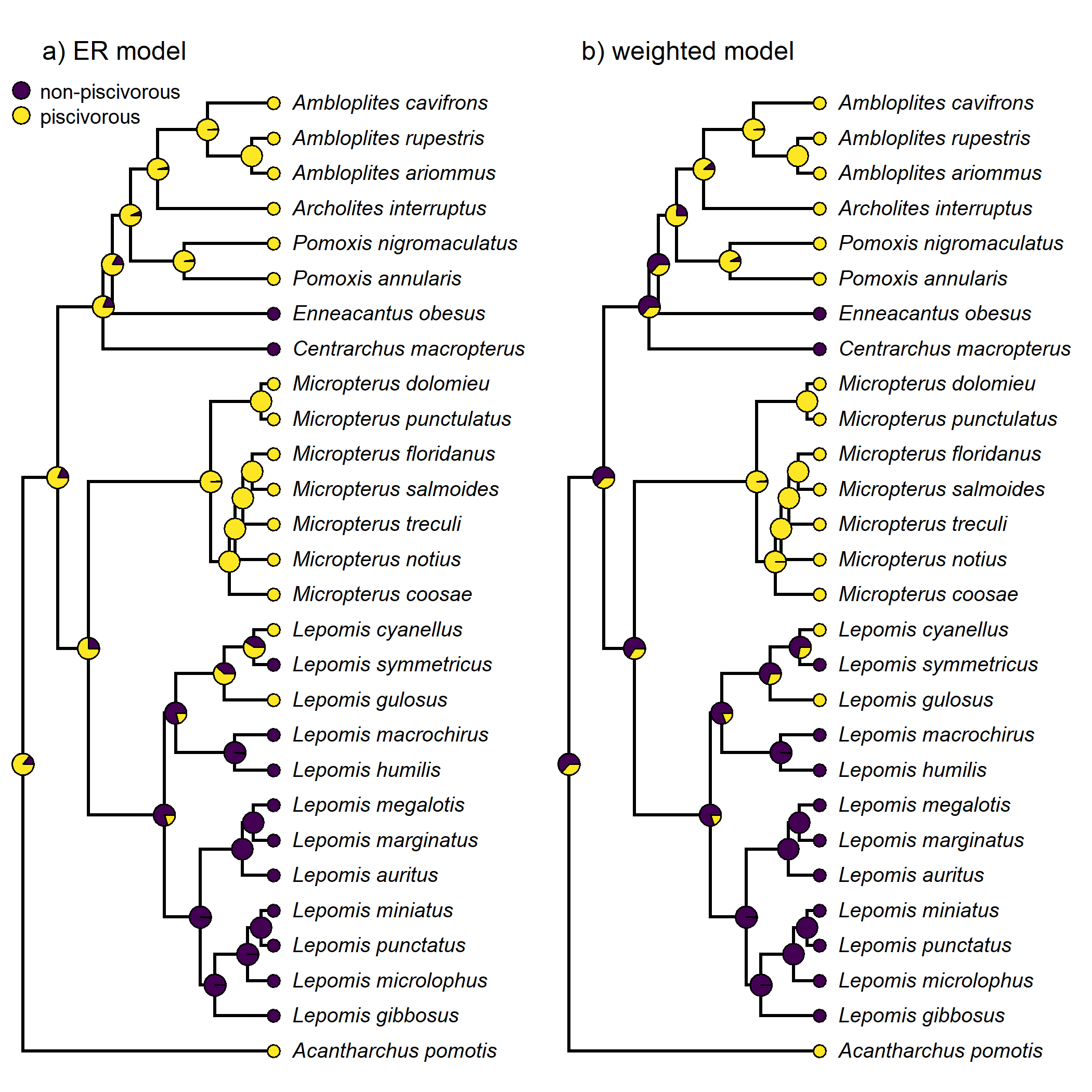## Sunday, February 19, 2023

### New generic stochastic mapping method for multiple fitted Mk discrete character model types in phytools

Inspired, to some degree, by recent updates to the phangorn R package by Klaus Schliep, I decided to add a new, still kind of experimental, generic `simmap` method to the phytools package.

Our normal stochastic character mapping (Huelsenbeck et al. 2003) workflow in phytools, and as illustrated in my recent book with Luke Harmon, might be to first fit a set of alternative discrete character evolution (Mk) models using (say) `fitMk`, choose the best-supported model under some criterion (perhaps AIC), generate a set of 100 or 1,000 stochastic character mappings of our trait under this model, and then summarize the results.

The purpose of the new generic method would be to take our model (or set of fitted models) from step one, and then just simply pass this object to the generic method, which would then spit back our stochastic character mapped trees! The principle is that this would make extending the generic to different model classes very easy – for instance, to an object of class `"fitpolyMk"` (from phytools’ polymorphic trait evolution model), `"fitPagel"`, or even `"fitHRM"` (for the hidden-rates model).

So far, I’ve created generic methods for two object classes: `"fitMk"` (the fitted model from `phytools::fitMk` for fitting the extended Mk model); and `"anova.fitMk"`.

The latter of these two is pretty neat because (by default) we can let the method sample stochastic character histories with relatively frequencies governed by the Akaike weights of each of our alternative models!

Here’s a very quick demo using the evolution of the character “parental care” (which has two different levels: `"male"` and `"none"`, but I’m going to re-name these), on a phylogenetic tree of bony fish species from Benun Sutton & Wilson (2019).

``````library(phytools)
packageVersion("phytools")
``````
``````##  '1.5.3'
``````

``````data(bonyfish.tree)
data(bonyfish.data)
``````

Separate out our character of interest.

``````parental_care<-setNames(bonyfish.data\$paternal_care,
rownames(bonyfish.data))
levels(parental_care)
``````
``````##  "male" "none"
``````

Re-name our levels.

``````levels(parental_care)<-c("paternal care","none")
``````

Now we can start just by fitting a single model, say the “all-rates-different” (`"ARD"`) model using `fitMk` and then trying to pass that to the new generic.

``````ARD.parental_care<-fitMk(bonyfish.tree,parental_care,
model="ARD",pi="fitzjohn")
ARD.parental_care
``````
``````## Object of class "fitMk".
##
## Fitted (or set) value of Q:
##               paternal care      none
## paternal care      0.000000  0.000000
## none               0.002229 -0.002229
##
## Fitted (or set) value of pi:
## paternal care          none
##             0             1
## due to treating the root prior as (a) nuisance.
##
## Log-likelihood: -31.542749
##
## Optimization method used was "nlminb"
``````
``````ard_trees.parental_care<-simmap(ARD.parental_care)
ard_trees.parental_care
``````
``````## 100 phylogenetic trees with mapped discrete characters
``````

Our next step, summarizing our findings, will depend (of course) on the purpose of our analyses. Here, I’ll use the phytools function `densityMap` to project the posterior probability of being in each of our two states along the edges & nodes of the tree.

``````dmap.parental_care<-densityMap(ard_trees.parental_care,
plot=FALSE)
``````
``````## sorry - this might take a while; please be patient
``````
``````## update color palette
COLS<-viridisLite::viridis(n=10)
dmap.parental_care<-setMap(dmap.parental_care,COLS)
plot(dmap.parental_care,fsize=c(0.4,0.9),outline=TRUE,lwd=c(2,4),
legend=150)
``````Next, let’s try the other generic `simmap` method that I’ve implemented so far – for objects of class `"anova.fitMk"`. These will be the result of calling the generic `anova` on a set of fitted Mk models from `fitMk`.

For this example, I’ll use feeding mode from a set of centrarchid fishes with data from Revell & Collar (2009).

``````data(sunfish.tree)
data(sunfish.data)
feeding_mode<-setNames(sunfish.data\$feeding.mode,
rownames(sunfish.data))
levels(feeding_mode)
``````
``````##  "non"  "pisc"
``````
``````## rename levels
levels(feeding_mode)<-c("non-piscivorous",
"piscivorous")
``````

Because we have two different levels of the trait, there are really only four possible models: equal-rates, different rates, and the two alternative directional models.

Let’s fit them.

``````ER.model<-fitMk(sunfish.tree,feeding_mode,
pi="fitzjohn")
irr.model1<-fitMk(sunfish.tree,feeding_mode,
model=matrix(c(0,1,0,0),2,2,byrow=TRUE),
pi="fitzjohn")
irr.model2<-fitMk(sunfish.tree,feeding_mode,
model=matrix(c(0,0,1,0),2,2,byrow=TRUE),
pi="fitzjohn")
ARD.model<-fitMk(sunfish.tree,feeding_mode,
model="ARD",pi="fitzjohn")
``````

To compare the four models, I’m going to use a generic `anova` method – but I’ll save the object it creates to a new object, here called `aov`.

``````aov<-anova(ER.model,irr.model1,irr.model2,ARD.model)
``````
``````##               log(L) d.f.      AIC    weight
## ER.model   -12.93221    1 27.86442 0.2409918
## irr.model1 -12.29505    1 26.59010 0.4557392
## irr.model2 -13.50717    1 29.01435 0.1356120
## ARD.model  -12.29505    2 28.59010 0.1676571
``````

Now the task of running our stochastic mapping is highly simplified. We’re just going to pass our `anova` result to our new `simmap` generic method and it’ll automatically sample character transition models from the four in our object, with probabilities equal to each model’s Akaike weight!

``````weighted_trees<-simmap(aov,nsim=1000)
weighted_trees
``````
``````## 1000 phylogenetic trees with mapped discrete characters
``````

Here are my 1,000 sampled trees.

``````cols<-setNames(COLS[c(1,length(COLS))],levels(feeding_mode))
par(mfrow=c(32,32))
plot(weighted_trees,colors=cols,lwd=1,ftype="off")
``````Lastly, let’s compare the posterior probabilities at nodes under this weighted mixture of different trait evolution models to the probabilities obtained if we (say) fit just a simple `"ER"` model.

``````par(mfrow=c(1,2))
plot(summary(simmap(ER.model,nsim=1000)),colors=cols,fsize=0.8,
ftype="i",cex=c(1,0.6),mar=c(0.1,0.1,2.1,0.1))